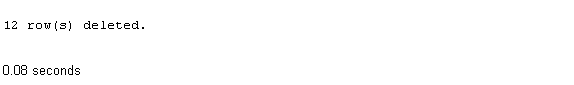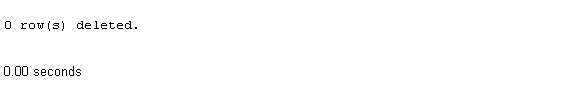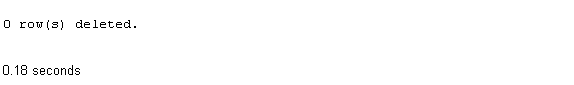# SQL Delete records using subqueries

## SQL Deleting records with subqueries

In this page, we are going to discuss, how SUBQUERIES (A SELECT statement within another SELECT statement can be used as a subquery )along with SQL DELETE command can be used to perform a deletion.

Sample table: customer1

Sample table: agents

Sample table: customer

Sample table: agent1

Sample table: orders

Example:

To remove rows from the table 'customer1' with following conditions -

1. 'agent_code' should be any 'agent_code' from 'agents' table which satisfies the condition bellow :

2. 'working_area' of 'agents' table must be 'London',

the following SQL statement can be used:

SQL Code:

``````DELETE FROM customer1
WHERE agent_code=ANY(
SELECT agent_code FROM agents
WHERE working_area='London');``````

Output:## SQL delete records using subqueries with alias

In this page, we are going to discuss, how table aliases( when two or more tables used in a query, then alias makes it easy to read and write with a short name which comes after the table name after the FROM keyword) can be used with SUBQUERIES (A SELECT statement within another SELECT statement can be used as a subquery ), and with the help of subqueries SQL DELETE command can be used to delete records.

Example:

To remove rows from the table 'agent1' with following conditions -

1. 'da' and 'cu' are the aliases for the table 'agent1' and 'customer'

2. check the existence of the subquery is true or false. which satisfies the condition bellow :

3. 'grade' of 'customer' table must be 3,

4. 'agent_code' of 'agent1' table and 'agent_code' of 'customer' table should not be same,

the following SQL statement can be used:

SQL Code:

``````DELETE FROM agent1 da
WHERE EXISTS(
SELECT * FROM  customer cu
AND da.agent_code<>cu.agent_code);
``````

Output:## SQL delete records using subqueries with alias and IN

In this page we are going to discuss, how rows can be removed from a table by SQL DELETE statement with the use of IN operator and SUBQUERIES.

Example:

To remove rows from the table 'agent1' with following conditions -

1. 'da' and 'cu' are the aliases of 'agent1' and 'customer' table,

2. check the number 3 is in the result of the subquery which satisfies the condition bellow :

3. 'agent_code' of 'agent1' table and 'agent_code' of 'customer' table should not be same,

the following SQL statement can be used:

SQL Code:

``````DELETE FROM agent1 da
WHERE 3 IN(
WHERE agent1.agent_code<>customer.agent_code);
``````

Output:## SQL delete records using subqueries with alias and MIN

In this page, we are going to discuss, how rows can be removed from a table by SQL DELETE statement along with the SQL MIN() function.

Example:

To remove rows from the table 'agent1' with following conditions -

1. 'orders' table used as alias 'a' and alias 'b',

2. 'agent_code' of 'agent1' should be within the 'agent_code' in alias 'a' which satisfies the condition bellow :

i) 'ord_amount' of alias 'a' must be equal to the minimum 'ord_amount' of alias 'b' which satisfies the condition bellow :

a) 'ord_date' of alias 'a' and alias 'b' must be equal,

the following SQL statement can be used :

SQL Code:

``````DELETE FROM agent1
WHERE agent_code IN
(SELECT agent_code FROM orders a
WHERE ord_amount=(
SELECT MIN(ord_amount) FROM orders b
WHERE a.ord_date=b.ord_date));``````

Output:## SQL delete records using subqueries with alias and MIN and COUNT

In this page, we are going to discuss, how rows can be removed from a table by SQL DELETE statement along with the SQL MIN() and COUNT() function.

Example:

To remove rows from the table 'agent1' with following conditions -

1. 'orders' table used as alias 'a' and alias 'b'

2. 'agent_code' of 'agent1' should be within the 'agent_code' in alias 'a' which satisfies the condition bellow:

i) 'ord_amount' of alias 'a' must be equal to the minimum 'ord_amount' of alias 'b' which satisfies the condition bellow :

a) 'ord_date' of alias 'a' and alias 'b' must be equal

ii) the number 1 should be less than the number of 'ord_num' form alias 'b' which satisfies the condition bellow :

a) 'ord_date' of alias 'a' and alias 'b' must be equal,

the following SQL statement can be used :

SQL Code:

``````DELETE FROM agent1
WHERE agent_code IN(
SELECT agent_code FROM orders a
WHERE ord_amount=(
SELECT MIN(ord_amount) FROM orders b
WHERE a.ord_date=b.ord_date)
AND 1<(
SELECT COUNT(ord_num) FROM orders b
WHERE a.ord_date=b.ord_date));
``````

Output:Outputs of the said SQL statement shown here is taken by using Oracle Database 10g Express Edition.

See our Model Database

Practice SQL Exercises